Feedback

# Standard error of measurement

### This is an excerpt from Statistics in Kinesiology 5th Edition With Web Resource by Joseph Weir & William Vincent.

The intraclass correlation coefficient provides an estimate of the relative error of the measurement; that is, it is unitless and is sensitive to the between-subjects variability. Because the general form of the intraclass correlation coefficient is a ratio of variabilities (see equation 13.04), it is reflective of the ability of a test to differentiate between subjects. It is useful for assessing sample size and statistical power and for estimating the degree of correlation attenuation. As such, the intraclass correlation coefficient is helpful to researchers when assessing the utility of a test for use in a study involving multiple subjects. However, it is not particularly informative for practitioners such as clinicians, coaches, and educators who wish to make inferences about individuals from a test result.

For practitioners, a more useful tool is the standard error of measurement (SEM; not to be confused with the standard error of the mean). The standard error of measurement is an absolute estimate of the reliability of a test, meaning it has the units of the test being evaluated and is not sensitive to the between-subjects variability of the data. Further, the standard error of measurement is an index of the precision of the test, or the trial-to-trial noise of the test. Standard error of measurement can be estimated with two common formulas. The first formula is the most common and estimates the standard error of measurement as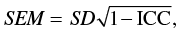(13.06)

where ICC is the intraclass correlation coefficient as described previously and SD is the standard deviation of all the scores about the grand mean. The standard deviation can be calculated quickly from the repeated measures ANOVA as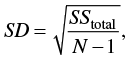(13.07)

where N is the total number of scores.

Because the intraclass correlation coefficient can be calculated in multiple ways and is sensitive to between-subjects variability, the standard error of measurement calculated using equation 13.06 will vary with these factors. To illustrate, we use the example data presented in table 13.5 and ANOVA summary from table 13.6. First, the standard deviation is calculated from equation 13.07 as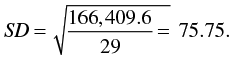Recall that we calculated ICC (1,1) = .30, ICC (2,1) = .40, and ICC (3,1) = .73. The respective standard error of measurement values calculated using equation 13.07 are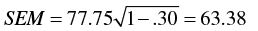for ICC (1,1),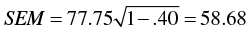for ICC (2,1),

and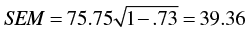for ICC (3,1).

Notice that standard error of measurement value can vary markedly depending on the magnitude of the intraclass correlation coefficient used. Also, note that the higher the intraclass correlation coefficient, the smaller the standard error of measurement. This should be expected because a reliable test should have a high reliability coefficient, and we would further expect that a reliable test would have little trial-to-trial noise and therefore the standard error should be small. However, the large differences between standard error of measurement estimates depending on which intraclass correlation coefficient value is used are a bit unsatisfactory.

Instead, we recommend using an alternative approach to estimating the standard error of measurement: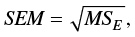(13.08)

where MSE is the mean square error term from the repeated measures ANOVA. From table 13.6, MSE = 1,044.54. The resulting standard error of measurement is calculated as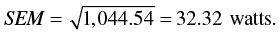This standard error of measurement value does not vary depending on the intraclass correlation coefficient model used because the mean square error is constant for a given set of data. Further, the standard error of measurement from equation 13.08 is not sensitive to the between-subjects variability. To illustrate, recall that the data in table 13.7 were created by modifying the data in table 13.1 such that the between-subjects variability (larger standard deviations) was increased but the means were unchanged. The mean square error term for the data in table 13.1 (see table 13.2, MSE= 1,070.28) was unchanged with the addition of between-subjects variability (see table 13.8). Therefore, the standard error of measurement values for both data sets are identical when using equation 13.08: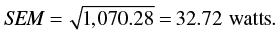### Interpreting the Standard Error of Measurement

As noted previously, the standard error of measurement differs from the intraclass correlation coefficient in that the standard error of measurement is an absolute index of reliability and indicates the precision of a test. The standard error of measurement reflects the consistency of scores within individual subjects. Further, unlike the intraclass correlation coefficient, it is largely independent of the population from which the results are calculated. That is, it is argued to reflect an inherent characteristic of the test, irrespective of the subjects from which the data were derived.

The standard error of measurement also has some uses that are especially helpful to practitioners such as clinicians and coaches. First, it can be used to construct a confidence interval about the test score of an individual. This confidence interval allows the practitioner to estimate the boundaries of an individual's true score. The general form of this confidence interval calculation is

T = S &plusmn; Zcrit (SEM), (13.09)

where T is the subject's true score, S is the subject's score on the test, and Zcrit is the critical Z score for a desired level of confidence (e.g., Z = 1.96 for a 95% CI). Suppose that a subject's observed score (S) on the Wingate test is 850 watts. Because all observed scores include some error, we know that 850 watts is not likely the subject's true score. Assume that the data in table 13.7 and the associated ANOVA summary in table 13.8 are applicable, so that the standard error of measurement for the Wingate test is 32.72 watts as shown previously. Using equation 13.09 and desiring a 95% CI, the resulting confidence interval is

T = 850 watts &plusmn; 1.96 (32.72 watts) = 850 &plusmn; 64.13 watts = 785.87 to 914.13 watts.

Therefore, we would infer that the subject's true score is somewhere between approximately 785.9 and 914.1 watts (with a 95% LOC). This process can be repeated for any subsequent individual who performs the test.

It should be noted that the process described using equation 13.09 is not strictly correct, and a more complicated procedure can give a more accurate confidence interval. For more information, see Weir (2005). However, for most applications the improved accuracy is not worth the added computational complexity.

A second use of the standard error of measurement that is particularly helpful to practitioners who need to make inferences about individual athletes or patients is the ability to estimate the change in performance or minimal difference needed to be considered real (sometimes called the minimal detectable change or the minimal detectable difference). This is typical in situations in which the practitioner measures the performance of an individual and then performs some intervention (e.g., exercise program or therapeutic treatment). The test is then given after the intervention, and the practitioner wishes to know whether the person really improved. Suppose that an athlete improved performance on the Wingate test by 100 watts after an 8-week training program. The savvy coach should ask whether an improvement of 100 watts is a real increase in anaerobic fitness or whether a change of 100 watts is within what one might expect simply due to the measurement error of the Wingate test. The minimal difference can be estimated as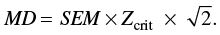(13.10)

Again, using the previous value of SEM = 32.72 watts and a 95% CI, the minimal difference value is estimated to be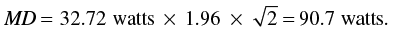We would then infer that a change in individual performance would need to be at least 90.7 watts for the practitioner to be confident, at the 95% LOC, that the change in individual performance was a real improvement. In our example, we would be 95% confident that a 100-watt improvement is real because it is more than we would expect just due to the measurement error of the Wingate test. Hopkins (2000) has argued that the 95% LOC is too strict for these types of situations and a less severe level of confidence should be used. This is easily done by choosing a critical Z score appropriate for the desired level of confidence.

It is not intuitively obvious why the use of the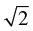term in equation 13.10 is necessary. That is, one might think that simply using equation 13.09 to construct the true score confidence interval bound around the preintervention score and then seeing whether the postintervention score is outside that bound would provide the answer we seek. However, this argument ignores the fact that both the preintervention score and the postintervention score are measured with error, and this approach considers only the measurement error in the preintervention score. Because both observed scores were measured with error, simply observing whether the second score falls outside the confidence interval of the first score does not account for both sources of measurement error.

We use theterm because we want an index of the variability of the difference scores when we calculate the minimal difference. The standard deviation of the difference scores (SDd) provides such an index, and when there are only two measurements like we have here,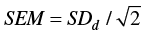We can then solve for the standard deviation of the difference scores by multiplying the standard error of measurement by. Equation 13.10 can be reconceptualized as

MD = SDd &times; Zcrit.

As with equation 13.09, the approach outlined in equation 13.10 is not strictly correct, and a modestly more complicated procedure can give a slightly more accurate confidence interval. However, for most applications the procedures described are sufficient.

An additional way to interpret the size of the standard error of measurement is to convert it to a type of coefficient of variation (CoV). Recall from chapter 5 that we interpreted the size of a standard deviation by dividing it by the mean and then multiplying by 100 to convert the value to a percentage (see equation 5.05). We can perform a similar operation with the standard error of measurement as follows: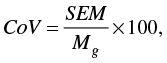(13.11)

where CoV = the coefficient of variation, SEM = the standard error of measurement, and MG = the grand mean from the data. The resulting value expresses the typical variation as a percentage (Lexell and Downham, 2005). For the example data in table 13.7 and the associated ANOVA summary in table 13.8, SEM = 32.72 watts (as shown previously) and MG = 774.0 (calculations not shown). The resulting CoV = 32.72/774.0 &times; 100 = 4.23%. This normalized standard error of measurement allows researchers to compare standard error of measurement values between different tests that have different units, as well as to judge how big the standard error of measurement is for a given test being evaluated.